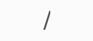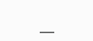# How do I input numbers and variables in the Advanced mode?

### Basic input rules for whole numbers, fractions, mixed numbers, and decimals.

To enter numbers, use the number entry keys, including "." for decimals and "/" and "_" for fractions.

In some cases, negative values should be entered in parentheses. Example: 2x*(-4_5/8), 2 + 7.2*(-5x).

##### Whole numbers.
To input whole numbers, use the corresponding number entry keys on the calculator keyboard.

##### Fractions.
Fractions are typed usingas the fraction bar.

So, "two-thirds" would be typed as 2/3.

"One-half" would be typed as 1/2.

Fractions can be entered with or without parentheses.

##### Mixed fractionssymbol is used to separate the whole number and the fraction.

So, "three and one half" would be typed as 3_1/2.

##### Decimal numbers
Use a decimal point to separate the whole part from the decimal part (ex. 12.34, 0.4 or .4).

Friday, September 22, 2023

Contact